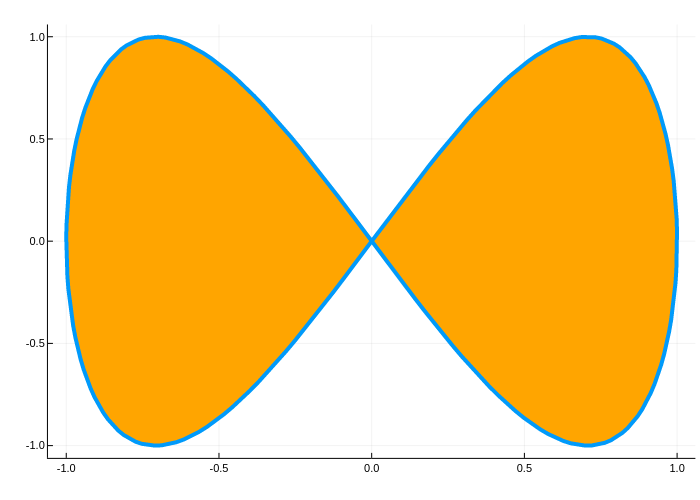# Parametric plotsusing Plots
plotlyjs()

Plot function pair (x(u), y(u)).

plot(sin, (x->begin
sin(2x)
end), 0, 2π, line = 4, leg = false, fill = (0, :orange))